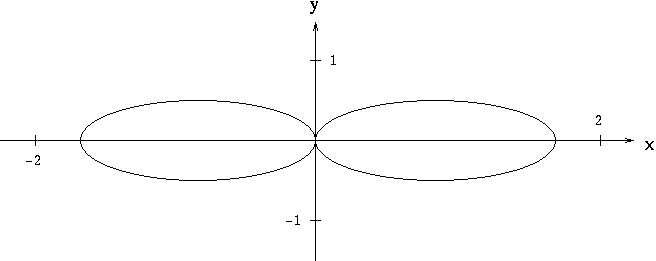IMPLICIT DIFFERENTIATION PROBLEMS

The following problems require the use of implicit differentiation. Implicit differentiation is nothing more than a special case of the well-known chain rule for derivatives. The majority of differentiation problems in first-year calculus involve functions y written EXPLICITLY as functions of x . For example, if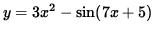,

then the derivative of y is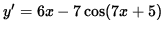.

However, some functions y are written IMPLICITLY as functions of x . A familiar example of this is the equation

x2 + y2 = 25 ,

which represents a circle of radius five centered at the origin. Suppose that we wish to find the slope of the line tangent to the graph of this equation at the point (3, -4) .How could we find the derivative of y in this instance ? One way is to first write y explicitly as a function of x . Thus,

x2 + y2 = 25 ,

y2 = 25 - x2 ,

and,

where the positive square root represents the top semi-circle and the negative square root represents the bottom semi-circle. Since the point (3, -4) lies on the bottom semi-circle given by,

the derivative of y is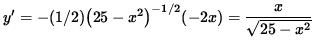,

i.e.,.

Thus, the slope of the line tangent to the graph at the point (3, -4) is.

Unfortunately, not every equation involving x and y can be solved explicitly for y . For the sake of illustration we will find the derivative of y WITHOUT writing y explicitly as a function of x . Recall that the derivative (D) of a function of x squared, (f(x))2 , can be found using the chain rule :.

Since y symbolically represents a function of x, the derivative of y2 can be found in the same fashion :.

Now begin with

x2 + y2 = 25 .

Differentiate both sides of the equation, getting

D ( x2 + y2 ) = D ( 25 ) ,

D ( x2 ) + D ( y2 ) = D ( 25 ) ,

and

2x + 2 y y' = 0 ,

so that

2 y y' = - 2x ,

and,

i.e.,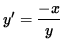.

Thus, the slope of the line tangent to the graph at the point (3, -4) is.

This second method illustrates the process of implicit differentiation. It is important to note that the derivative expression for explicit differentiation involves x only, while the derivative expression for implicit differentiation may involve BOTH x AND y .

The following problems range in difficulty from average to challenging.

• PROBLEM 1 : Assume that y is a function of x . Find y' = dy/dx for x3 + y3 = 4 .

• PROBLEM 2 : Assume that y is a function of x . Find y' = dy/dx for (x-y)2 = x + y - 1 .

• PROBLEM 3 : Assume that y is a function of x . Find y' = dy/dx for.

• PROBLEM 4 : Assume that y is a function of x . Find y' = dy/dx for y = x2 y3 + x3 y2 .

• PROBLEM 5 : Assume that y is a function of x . Find y' = dy/dx for exy = e4x - e5y .

• PROBLEM 6 : Assume that y is a function of x . Find y' = dy/dx for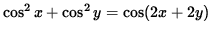.

• PROBLEM 7 : Assume that y is a function of x . Find y' = dy/dx for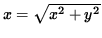.

• PROBLEM 8 : Assume that y is a function of x . Find y' = dy/dx for.

• PROBLEM 9 : Assume that y is a function of x . Find y' = dy/dx for.

• PROBLEM 10 : Find an equation of the line tangent to the graph of (x2+y2)3 = 8x2y2 at the point (-1, 1) .

• PROBLEM 11 : Find an equation of the line tangent to the graph of x2 + (y-x)3 = 9 at x=1 .

• PROBLEM 12 : Find the slope and concavity of the graph of x2y + y4 = 4 + 2x at the point (-1, 1) .

• PROBLEM 13 : Consider the equation x2 + xy + y2 = 1 . Find equations for y' and y'' in terms of x and y only.

• PROBLEM 14 : Find all points (x, y) on the graph of x2/3 + y2/3 = 8 (See diagram.) where lines tangent to the graph at (x, y) have slope -1 .• PROBLEM 15 : The graph of x2 - xy + y2 = 3 is a "tilted" ellipse (See diagram.). Among all points (x, y) on this graph, find the largest and smallest values of y . Among all points (x, y) on this graph, find the largest and smallest values of x .• PROBLEM 16 : Find all points (x, y) on the graph of (x2+y2)2 = 2x2-2y2 (See diagram.) where y' = 0.